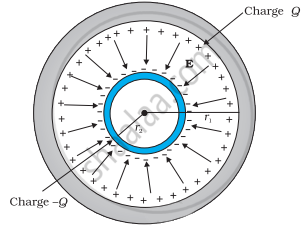# A spherical capacitor consists of two concentric spherical conductors, held in position by suitable insulating supports. Show that the capacitance of a - Physics

Numerical

A spherical capacitor consists of two concentric spherical conductors, held in position by suitable insulating supports. Show that the capacitance of a spherical capacitor is given by

C = (4piin_0"r"_1"r"_2)/("r"_1 - "r"_2)where r1 and r2 are the radii of outer and inner spheres, respectively.

#### Solution

Radius of the outer shell = r1

Radius of the inner shell = r2

The inner surface of the outer shell has charge +Q.

The outer surface of the inner shell has induced charge −Q.

Potential difference between the two shells is given by,

"V" = "Q"/(4piin_0"r"_2) - "Q"/(4piin_0"r"_1)

Where,

in_0 = Permittivity of free space

"V" ="Q"/(4piin_0)[1/"r"_2 - 1/"r"_1]

= ("Q"("r"_1 - "r"_2))/(4piin_0"r"_1"r"_2]

Capacitance of the given system is given by

C = "Charge (Q)"/"Potenstial difference (V)"

= (4piin_0"r"_1"r"_2)/("r"_1 - "r"_2)

Hence, proved.

Concept: Capacitors and Capacitance
Is there an error in this question or solution?

#### APPEARS IN

NCERT Physics Part 1 and 2 Class 12
Chapter 2 Electrostatic Potential and Capacitance
Exercise | Q 2.29 | Page 90
NCERT Class 12 Physics Textbook
Chapter 2 Electrostatic Potential and Capacitance
Exercise | Q 29 | Page 90

Share